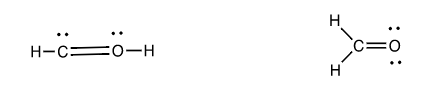# Problem: The formaldehyde molecule, which has the formula CH 2O, has two possible structures for which we can write a correct Lewis dot structures.  Using formal charge, determine the correct structure.  Be sure to show how you compute the formal charge on each atom.

###### FREE Expert Solution
95% (308 ratings)
###### Problem Details

The formaldehyde molecule, which has the formula CH 2O, has two possible structures for which we can write a correct Lewis dot structures.  Using formal charge, determine the correct structure.  Be sure to show how you compute the formal charge on each atom.